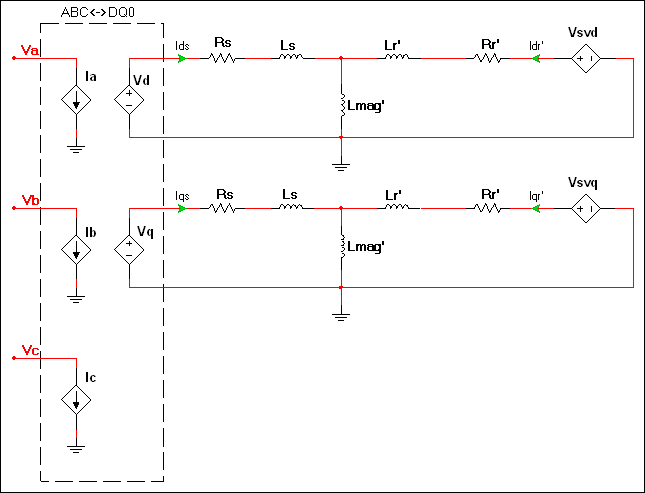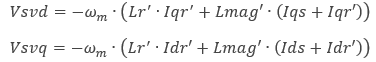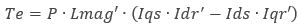OK

# Induction machine squirrel cage model

Model assumptions

• Linear iron magnetization with no saturation
• Uniform air-gap
• No slot harmonics
• Sinusoidally distributed windings
• No zero phase sequence (system is balanced)
Configuration of the machine electrical systemwhere

ωm = mechanical angular speed

The elements in the dashed region represent a bidirectional ABC<->DQ0 coordinate transform in the stationary reference frame. Refer to DQ0 coordinate transforms for representative equations.

The generated electromagnetic torque is as follows:where

P = Number of pair poles

Parameter Description
Stator leakage Ls in the diagram of the component’s electrical system.
Stator resistance Rs in the diagram of the component’s electrical system.
Rotor leakage inductance Lr' in the Vsvd and Vsvq equations.
Rotor resistance Rr' in the diagram of the component’s electrical system.
Magnetizing inductance Lmag' in the Vsvd and Vsvq equations.
Number of pole pairs P in the equation for generated electromagnetic torque.
Shaft inertia J<sub>rotor</sub> on the machine model diagram in <a href="/help/components/machine-modeling/">Machine modeling</a>.
Shaft friction This is F<sub>rotor</sub> on the machine model diagram in <a href="/help/components/machine-modeling/">Machine modeling</a>.
Initial angular speed Rotational measurement of the shaft angle in rad/s at the start of the simulation.